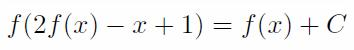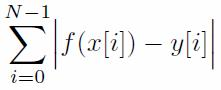### Problem Statement

f is a function from integers to integers. In other words, f is defined over integers, and f(x) is an integer for all integers x. You are given an integer C. f is called C-beautiful if the following equality is satisfied for all integers x:Return the minimal possible value of the following formula when f is C-beautiful:Use the following recursive definitions to generate the sequences x and y:
• x = xzero
• For all integer i between 1 and N-1, inclusive, x[i] = (x[i-1] * xprod + xadd) % xmod
• y = yzero
• For all integer i between 1 and N-1, inclusive, y[i] = (y[i-1] * yprod + yadd) % ymod

### Definition

 Class: FunctionalEquation Method: minAbsSum Parameters: int, int, int, int, int, int, int, int, int, int Returns: long Method signature: long minAbsSum(int C, int N, int xzero, int xprod, int xadd, int xmod, int yzero, int yprod, int yadd, int ymod) (be sure your method is public)

### Notes

-64-bit integers should be used to generate the sequences x and y to avoid overflow.

### Constraints

-C will be between 1 and 16, inclusive.
-N will be between 1 and 10,000, inclusive.
-xmod and ymod will each be between 1 and 1,000,000,000, inclusive.
-xzero, xprod and xadd will each be between 0 and xmod - 1, inclusive.
-yzero, yprod and yadd will each be between 0 and ymod - 1, inclusive.

### Examples

0)

 `3` `10` `0` `1` `1` `456` `1` `1` `1` `456`
`Returns: 0`
 f(x) = x + 1 is a 3-beautiful function. Since x = {0, 1, 2, 3, 4, 5, 6, 7, 8, 9} and y = {1, 2, 3, 4, 5, 6, 7, 8, 9, 10}, the sum of |f(x[i]) - y[i]| is 0.
1)

 `4` `10` `0` `1` `1` `456` `1` `1` `1` `456`
`Returns: 5`
 x and y are the same as in example 0, but f(x) = x + 1 is not a 4-beautiful function.
2)

 `16` `10000` `654816386` `163457813` `165911619` `987654321` `817645381` `871564816` `614735118` `876543210`
`Returns: 3150803357206`

#### Problem url:

http://www.topcoder.com/stat?c=problem_statement&pm=10570

#### Problem stats url:

http://www.topcoder.com/tc?module=ProblemDetail&rd=13909&pm=10570

rng_58

#### Testers:

PabloGilberto , misof , ivan_metelsky

#### Problem categories:

Graph Theory, Math